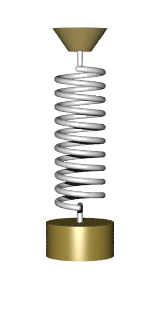You Might LikeAn undamped spring–mass system is an oscillatory system

Oscillation is the repetitive variation, typically in time, of some measure about a central value (often a point of equilibrium) or between two or more different states. The term vibration is precisely used to describe mechanical oscillation. Familiar examples of oscillation include a swinging pendulum and alternating current.

Oscillations occur not only in mechanical systems but also in dynamic systems in virtually every area of science: for example the beating of the human heart (for circulation), business cycles in economics, predator–prey population cycles in ecology, geothermal geysers in geology, vibration of strings in guitar and other string instruments, periodic firing of nerve cells in the brain, and the periodic swelling of Cepheid variable stars in astronomy.

# Simple harmonic

The simplest mechanical oscillating system is a weight attached to a linear spring subject to only weight and tension. Such a system may be approximated on an air table or ice surface. The system is in an equilibrium state when the spring is static. If the system is displaced from the equilibrium, there is a net restoring force on the mass, tending to bring it back to equilibrium. However, in moving the mass back to the equilibrium position, it has acquired momentum which keeps it moving beyond that position, establishing a new restoring force in the opposite sense. If a constant force such as gravity is added to the system, the point of equilibrium is shifted. The time taken for an oscillation to occur is often referred to as the oscillatory period.

The systems where the restoring force on a body is directly proportional to its displacement, such as the dynamics of the spring-mass system, are described mathematically by the simple harmonic oscillator and the regular periodic motion is known as simple harmonic motion. In the spring-mass system, oscillations occur because, at the static equilibrium displacement, the mass has kinetic energy which is converted into potential energy stored in the spring at the extremes of its path. The spring-mass system illustrates some common features of oscillation, namely the existence of an equilibrium and the presence of a restoring force which grows stronger the further the system deviates from equilibrium.

# Damped and driven oscillations

All real-world oscillator systems are thermodynamically irreversible. This means there are dissipative processes such as friction or electrical resistance which continually convert some of the energy stored in the oscillator into heat in the environment. This is called damping. Thus, oscillations tend to decay with time unless there is some net source of energy into the system. The simplest description of this decay process can be illustrated by oscillation decay of the harmonic oscillator.

In addition, an oscillating system may be subject to some external force, as when an AC circuit is connected to an outside power source. In this case the oscillation is said to be driven.

Some systems can be excited by energy transfer from the environment. This transfer typically occurs where systems are embedded in some fluid flow. For example, the phenomenon of flutter in aerodynamics occurs when an arbitrarily small displacement of an aircraft wing (from its equilibrium) results in an increase in the angle of attack of the wing on the air flow and a consequential increase in lift coefficient, leading to a still greater displacement. At sufficiently large displacements, the stiffness of the wing dominates to provide the restoring force that enables an oscillation.

# Coupled oscillations

The harmonic oscillator and the systems it models have a single degree of freedom. More complicated systems have more degrees of freedom, for example two masses and three springs (each mass being attached to fixed points and to each other). In such cases, the behavior of each variable influences that of the others. This leads to a coupling of the oscillations of the individual degrees of freedom. For example, two pendulum clocks (of identical frequency) mounted on a common wall will tend to synchronise. This phenomenon was first observed by Christiaan Huygens in 1665. The apparent motions of the compound oscillations typically appears very complicated but a more economic, computationally simpler and conceptually deeper description is given by resolving the motion into normal modes.

More special cases are the coupled oscillators where energy alternates between two forms of oscillation. Well-known is the Wilberforce pendulum, where the oscillation alternates between an elongation of a vertical spring and the rotation of an object at the end of that spring.

Coupled oscillators is a common description of two related, but different phenomena. One case is where both oscillations affect each other mutually, which usually leads to the occurrence of a single, entrained oscillation state, where both oscillate with a compromise frequency. Another case is where one external oscillation affects an internal oscillation, but is not affected by this. In this case the regions of synchronization, known as Arnold tongues. The latter case can lead to highly complex phenomena as for instance chaotic dynamics.

# Continuous systems – waves

As the number of degrees of freedom becomes arbitrarily large, a system approaches continuity; examples include a string or the surface of a body of water. Such systems have (in the classical limit) an infinite number of normal modes and their oscillations occur in the form of waves that can characteristically propagate.

# Mathematics

The mathematics of oscillation deals with the quantification of the amount that a sequence or function tends to move between extremes. There are several related notions: oscillation of a sequence of real numbers, oscillation of a real valued function at a point, and oscillation of a function on an interval (or open set).